Download PresentationMetric based KNN indexing

# Metric based KNN indexing - PowerPoint PPT Presentation

Metric based KNN indexing. Lecturer : Prof Ooi Beng Chin Presenters : Frankie Chan HT00-3550Y Tan Zhenqiang HT01-6163J. Outline. Introduction Examples SR-Tree MVP-Tree Future works Conclusion References. Introduction.I am the owner, or an agent authorized to act on behalf of the owner, of the copyrighted work described.
Download Presentation## Metric based KNN indexing

An Image/Link below is provided (as is) to download presentation

Download Policy: Content on the Website is provided to you AS IS for your information and personal use and may not be sold / licensed / shared on other websites without getting consent from its author.While downloading, if for some reason you are not able to download a presentation, the publisher may have deleted the file from their server.

- - - - - - - - - - - - - - - - - - - - - - - - - - E N D - - - - - - - - - - - - - - - - - - - - - - - - - -
Presentation Transcript### Metric based KNN indexing

Lecturer : Prof Ooi Beng Chin

Presenters : Frankie Chan HT00-3550Y

Tan Zhenqiang HT01-6163J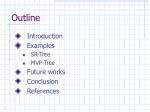Outline
• Introduction
• Examples
• SR-Tree
• MVP-Tree
• Future works
• Conclusion
• References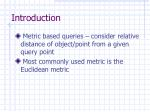Introduction
• Metric based queries – consider relative distance of object/point from a given query point
• Most commonly used metric is the Euclidean metricMetric based queries
• Variations :
• used with joins queries – find 3 closest restaurants for each of 2 different theaters
• used with spatial queries – find KNN to east of a location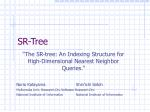### SR-Tree

“The SR-tree: An Indexing Structure for High-Dimensional Nearest Neighbor Queries.”

Norio Katayama Shin’ichi Satoh

Multimedia Info. Research Div. Software Research Div.

National Institute of Informatics National Institute of Informatics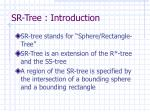SR-Tree : Introduction
• SR-tree stands for “Sphere/Rectangle-Tree”
• SR-Tree is an extension of the R*-tree and the SS-tree
• A region of the SR-tree is specified by the intersection of a bounding sphere and a bounding rectangle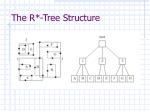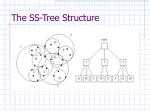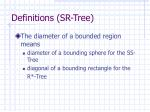Definitions (SR-Tree)
• The diameter of a bounded region means
• diameter of a bounding sphere for the SS-Tree
• diagonal of a bounding rectangle for the

R*-Tree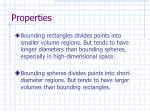Properties
• Bounding rectangles divides points into smaller volume regions. But tends to have longer diameters than bounding spheres, especially in high-dimensional space.
• Bounding spheres divides points into short-diameter regions. But tends to have larger volumes than bounding rectangles.Properties
• SR-Tree combined the use of bounding sphere and bounding rectangle, as the properties are complementary to each other.Indexing Structure
• The structure of the leaf L :
• The structure of the node N :Insertion Algorithm
• SR-tree insertion algorithm is based on

SS-tree’s centroid-based algorithm

• Descend down the tree and choose a

subtree with the centroid nearest to

the new entry

• SR-tree algorithm updates both

bounding spheres (diff. from SS-tree)

and bounding rectangles (same as

R*-tree)Insertion Algorithm
• Bounding sphere computation :
• Center, X (X1 ,X2 ,……..,XD)Deletion Algorithm
• SR-tree deletion algorithm is similar to

that of the R-tree

• If entry deletion do not cause

leaf/node under-utilisation, then just

remove it

• Otherwise, remove under-utilised

leaf/node and reinsert all orphaned

entriesNearest Neighbour Search
• Algorithm – ordered depth-first search
• It finds a number of points nearest to the

query, to make a candidate set

• Then it revises the candidate set, when it

visits every leaf whose region overlaps the

range of the candidate set

• After it visited all leave, the final candidate is

the search resultDefinition (NN search)
• Minimum Distance (MINDIST) euclidean distance from query point to the bounded region
• Minimax Distance (MINMAXDIST) minimum value of all the maximum distances between the query point and points on each n axes respectivelySearch pruning
• Region R1 with MINDIST greater than MINMAXDIST of another region R2 is discarded, because it cannot contain NN (downward pruning)
• Actual distance from query point P to a given object O which is greater than MINMAXDIST of a region, is discarded (upward pruning)
• Region with MINDIST greater than the actual distance from query point P to an object O, is discarded (upward pruning)Recursive procedure (leaf node)

If Node.type = LEAF then

For I := 1 to Node.count

dist := objectDist(Pt,Node.region)

if (dist < Nearest.dist) then

Nearest.dist := dist

Nearest.region := Node.regionRecursive procedure (non-leaf node)

Else /* non-leaf node => order, sort, prune & visit node */

genBranchList(Pt,Node,branchList)

sortBranchList(branchList)

/* perform downward pruning */

last = pruneBranch(Node,Pt,NearestBranchList)

For I := 1 to last

newNode := Node.branch

nearestNeighborSearch(newNode,Pt,Nearest)

/* perform upward pruning */

last := pruneBranchList(Node,Pt,Nearest,

branchList)Performance Analysis - Insertion

Insertion cost of R*-tree, SS-tree and SR-tree (uniform dataset)Performance Analysis - Query

Performance of VAMSplit R-tree, SS-tree and SR-tree

(Uniform dataset)Performance Analysis - Query

Performance of VAMSplit R-tree, SS-tree and SR-tree

(Real dataset)SR-tree average volume & diameter

Average volume & diameter of the leaf-level regions of R*-tree, SS-tree and SR-tree (real dataset)Strengths
• SR-Tree divides points into regions with small volumes and short diameter.
• Division of points into smaller regions improves disjointness.
• Smaller volume and diameter enhances the nearest neighbour queries’ performance.Weaknesses
• SR-tree suffers from the fanout problem (branching factor – max node entries)
• The node size grows as dimensionality increases
• The reduction of the fanout may requires more nodes to be read on queries
• Possibly affect query performance### MVP-Tree

“Indexing Large Metric Spaces For Similarity Search Queries”

Tolga Bozkaya Meral Ozsoyoglu

Oracle corporation Dept. Comp Eng & Sci

Case Western Reserve Univ.Outline
• Main idea.
• Algorithm basis
• How to build mvp-tree based on the given data.
• How to do similarity search in mvp-tree.
• Performance analysis and comparison based on experiments.Main Idea
• Triangle Inequality. Distance based Indexing
• Adopt more vantage points and levels
• Pre-computed distances are kept in leaf node.
• Use pre-computed distances to prune query branches.Algorithm Basis
• vp1,vp2,vp3 are vantage points.
• Q is given query point
• p1 belongs to points set
• The more vantage points the more unnecessary query branches are pruned.
• The distance between two vantage points is normally the larger the betterHow to construct mvp-tree(m,k,p)
• 1) If | S | = 0 then create an empty tree and quit.
• 2) If | S | £ k then Create leaf node L, put all data to L, Quit.
• 3) Choose first vantage point Svp1, Keep distances in arrays.
• 4) Divide S into m groups with same cardinalities based on the distances between Svp1 to points in S. And keeps distances as well.
• 5) For the first v above group
• 5.1) Choose last point in previous group as new vantage point.
• 5.2) Divide the group into m sub-groups with same cardinalities based on the distances between Svp1 to points in S. And keeps distances as well.
• 6) Recursively create mvp-tree on the mv sub-groups based on the steps from 1) to 5).Example
• Sv1:first vantage point(level 1)
• Sv2:vantange points(level 2)
• D[1..k]:the distances between

data points in leaf node and

vantage points

• x.Path[p]:the distances between

the data point and the first p vantage points along the path from the root to the leaf node that keeps itHow to do similarity search
• Depth-first process. Q is the given query object. r is the distance.
• 1) For i=1 to m
• If d(Q, Svi) £ r then Svi is in the answer set.

(Svi is the ith vantage points in current node.)

• 2) If current node is leaf node
• For all data points ( Si) in the node,

If for all vantage points Sv,

[d(Q, Sv) - r £ d(Si, Sv) £ d(Q, Sv) + r] holds, and

• for all i=1..p ( PATH[i] - r £ Si.PATH[i] £ PATH[i] + r ) holds,
• then compute d(Q, Si). If d(Q, Si) £ r, then Si is in the answer set.
• 3) If the current node is an internal node

for all i=1..m

if d(Q, Svi) + r £ Mi then recursively search the first branch

(Mi is the maximum of the distances between Svi to those points in its child node)Comparison Experiment
• Performance results for the queries with the data set where data points form several physical clustersPerformance Analysis
• Experiments to compare mvp-trees with vp-trees but use only one vantage point at each level, and do not make use of the pre-computed distances show that mvp-tree outperforms the vp-tree by 20% to 80% for varying query ranges and different distance distributions.
• For small query ranges, experiments with Euclidean vectors showed that mvp-trees require 40% to 80% less distance computation compared to vp-trees.
• For higher query ranges, the percentagewise difference decrease gradually, yet mvp-trees still perform better, making up to 30% less distance computations for the largest query ranges used.
• Experiments on gray-level images using the data set with 1151 images show mvp-trees performed up to 20-30% less distance computations.Strengths
• Based on the thoughts of triangle inequality, more vantage points more unnecessary query branches pruned, the longer distances among vantage points the better and reusing pre-computed distances as much as possible.
• Mvp-tree is flatter than vp-tree.
• It is also balanced because of the way it is constructed.
• Experiments show that it is more efficient than vp- tree and M-tree.Weaknesses

1.Construction cost O(nlogmn) distances computations

2.Additional storage cost are very high. There must be an array of size of p in every data point in leaf node.

3.Updating and inserting data points maybe lead to reconstruction of the mvp-tree.

4.If the insertions cause the tree structure to be skewed (that is, the additions of new data points change the distance distribution of the whole data set), global restructuring may have to be done, possibly during off hours of operation.

5.As the mvp-tree is created from an initial set of data objects in a top-down fashion, it is a rather static index structure.Conclusion
• metric based indexing can be effective for high dimensional and non-uniform datasets (eg. Image/video similarity indexing)
• future work :
• algorithm to perform in both dynamic & static database environment
• analyse the use of metric with other attributes to enable range queriesReferences
• N. Katayama and S. Satoh. The SR-tree: An Indexing Structure for High-Dimensional Nearest Neighbor Queries. Proc. ACM SIGMOD Int. Conf. on Management of Data, pages 369--380, Tucson, Arizona, 1997.
• R. Kurniawati, J. S. Jin, and J. A. Shepherd. The SS + -tree: An Improved Index Structure for Similarity Searches in a High-Dimensional Feature Space. SPIE: Storage and Retrieval for Image and Video Databases V , pages 110--120, 1997.
• N. Beckmann, H.P. Kriegel, R. Schneider, and B. Seeger. The R*tree : An Efcient and Robust Access Method for Points and Rectangles. In Proc. ACM SIGMOD Intl. Symp. on the Management of Data, pages 322--331, 1990.
• N. Roussopoulosi, S. Kelley, and F. Vincent. Nearest Neighbor Queries. Proc. ACM SIGMOD, San Jose, USA, pages 71-79, May 1995.
• Tolga Bozkaya and Meral Ozsoyoglu. Indexing Large Metric Spaces For Similarity Search Queries. Association for Computing Machinery transactions on Database System, pages 1-34, 1999.
• Roberto Figueira Santos Filho, Agma Traina, Caetano Traina Jr and Christos Faloutsos. Similarity Search Without Tears: The OMNI-Family Of All-Purpose Access Methods. ICDE 2001.